## Carlyle Circle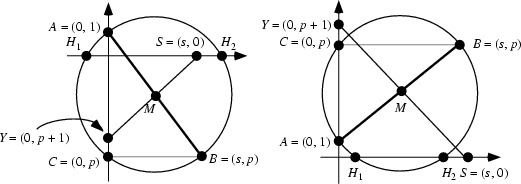Consider a Quadratic Equationwhereanddenote signed lengths. The Circle which has the pointsandas a Diameter is then called the Carlyle circleof the equation. The Center ofis then at the Midpoint of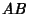,, which is also the Midpoint of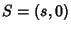and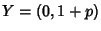. Call the points at whichcrosses the x-Axis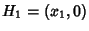and(with). Then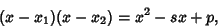soand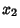are the Roots of the quadratic equation.

References

De Temple, D. W. Carlyle Circles and the Lemoine Simplicity of Polygonal Constructions.'' Amer. Math. Monthly 98, 97-108, 1991.

Eves, H. An Introduction to the History of Mathematics, 6th ed. Philadelphia, PA: Saunders, 1990.

Leslie, J. Elements of Geometry and Plane Trigonometry with an Appendix and Very Copious Notes and Illustrations, 4th ed., improved and exp. Edinburgh: W. & G. Tait, 1820.